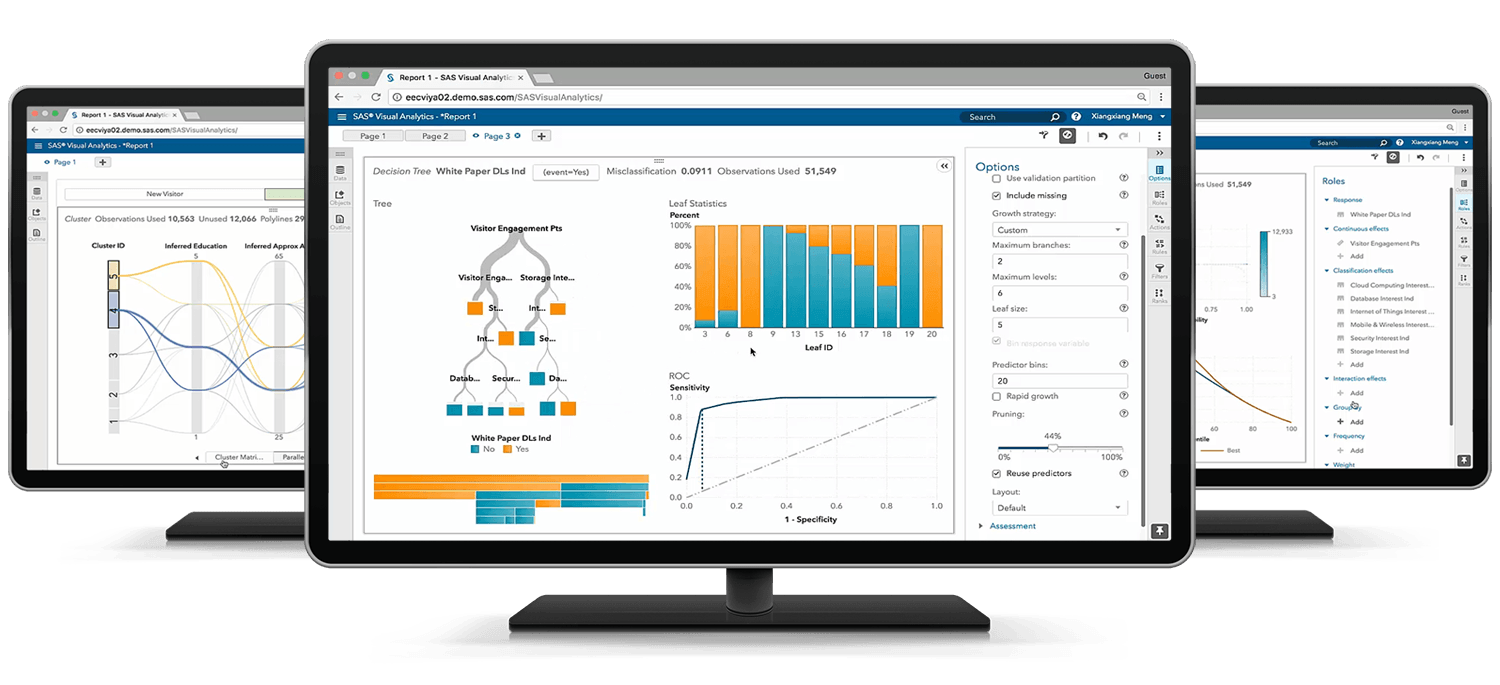# Statistical Thinking and Problem Solving

## Exam Content Guide

Below we provide a list of the objectives that will be tested on the exam.## Section 1: Statistical Thinking and Problem Solving: 10%

• Define statistical thinking and problem solving
• Define the process for creation of a process map or SIPOC
• Identify root causes of a problem, compile and collect data

## Section 2: Exploratory Data Analysis: 20%

• Describe data using descriptive statistics and graphical summaries
• Visualize and explore data
• Save and share results
• Prepare data for analysis

## Section 3: Quality Methods: 20%

• Use Statistical Process Control Charts
• Assess process capability
• Conduct Measurement System Studies

## Section 4: Decision Making with Data: 15%

• Define/describe Statistical Intervals
• Perform statistical tests
• Determine sample size

## Section 5: Correlation and Regression: 20%

• Be able to define and use correlation
• Define simple linear regression
• Define/explain multiple linear regression
• Define/explain logistic regression

## Section 6: Design of Experiments: 10%

• Define DOE and compare it to OFAT
• Discuss best practices and considerations to take when running an experiment
• Analyze the results of an experiment
• Design a simple experiment

## Section 7: Predictive Modeling and Text Mining: 5%

• Explain the difference between explanatory modeling versus predictive modeling
• Interpret results from a predictive model with validation